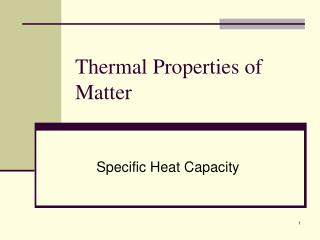DownloadDownload PresentationThermal Properties of Matter

# Thermal Properties of Matter

Télécharger la présentation## Thermal Properties of Matter

- - - - - - - - - - - - - - - - - - - - - - - - - - - E N D - - - - - - - - - - - - - - - - - - - - - - - - - - -
##### Presentation Transcript

1. Thermal Properties of Matter Specific Heat Capacity

2. Level: Secondary 3 Express (Pure Physics) • Duration: 2 periods (70 minutes) • Venue: Physics Laboratory

3. Lesson Objectives • Define the terms heat capacity and specific heat capacity. • Deduce the formula for heat capacity and specific heat capacity • Apply the above formulas to solve related problems. • Apply the knowledge of the high heat capacity of water to everyday applications.

4. Introduction Balloon filled with Air and Water Balloon filled with Air Candle A B

5. Question • If balloons A and B are heated by the candle, which one will burst? • Both balloon A and B will burst • Balloon A only • Balloon B only • None of them will burst • Support your answer with a reason. • Result: Only Balloon A will burst. Why? Stay tuned to find out!

6. Heat insulator Heat conductor Recall • Touch a metal spoon and a plastic spoon. Do you feel a difference? • Are they at the same temperature? • Good heat conductors conduct heat away from our skin more easily, making us feel cold. • Application: Frying pan

7. Expt 1A – Heating 20 ml water • Refer to “calorimetry.html” • Heat 20 ml of water, power 100W for 10 sec. • Note the initial and final temperature.

8. Expt 1B – Heating 40 ml water • Heat 40 ml of water, power 100W for 10 sec. • Note the initial and final temperature.

9. Thermal energy The ability to receive, hold, or absorb Heat capacity • For the same amount of heat energy supplied, different amounts of water have a different rise in temperature. • More water → rise in temperature is lower → need to supply more heat to achieve the same rise in temperature → higherheat capacity HeatCapacity

10. Experiment 2 • For the same amount of different substances, will they have different heat capacities? • Refer to Experiment 2 in worksheet VS Water Cooking Oil

11. Formal Definition • The same amount of heat supplied to different amounts of the same substance (water in this case) cause different rise in temperature. Likewise for same amounts of different substances. • How do we measure how much heat will raise the temperature by how much? Heat Capacity – The amount of heat energy needed to raise the temperature of a substance by 1 degree Celsius or 1 Kelvin

12. Formula of Heat Capacity • From definition: • Unit: J/K or J/oC • More useful form: • We write as

13. Exercises • When a piece of iron is heated from 30C to 60 C, the heat absorbed is 6000J. Calculate its heat capacity • A piece of copper has a heat capacity of 30 J/K. What is the amount of heat given out when it is cooled from 290 K to 200 K. • For experiments 1A and 1B, given that the heat capacity of the calorimeter is 53.64 J/K, calculate the respective heat capacity of water in both experiments.

14. Specific Heat Capacity • As observed from Expt 1A and 1B, since the heat capacity of water is dependent on the amount of water, it is more meaningful to look at heat capacity per unit mass of a substance. • The heat capacity of 1 g of the same substance (in this case water) is always the • same. This is called its specific heat capacity.

15. Specific Heat Capacity Specific Heat Capacity – The amount of heat energy needed to raise the temperature of 1 kg of substance by 1 degree Celsius or 1 Kelvin • From definition: • Unit: J/K or J/oC

16. Specific Heat Capacity • More useful form: Heat Supplied = Mass × Specific Heat Capacity × Change in Temperature • We write as

17. Exercises • Based on your observations in Experiment 2, what can we say about the specific heat capacities of water and oil? Explain why we use oil in cooking with regards to its specific heat capacity. • A piece of iron of mass 5 kg has a heat capacity of 2220 J K-1. Find its specific heat capacity. • Find the amount of heat energy needed to raise the temperature of 500g of water from 0oC to 100oC. Take the specific heat capacity of water to be 4186 J/kgoC.

18. Exercises • What are the applications that make use of the high specific heat capacity of water? • What are the differences between heat capacity and specific heat capacity of a substance?

19. Applications • Hot water bottle to keep warm on cold nights. • Water used as cooling liquid in car engines • Land and Sea breezes. • Others?

20. The balloon is stretched very thin by air and water. Due to the high heat capacity of water, heat from the candle is quickly absorbed by the water and the temperature does not rise high enough to melt the balloon. So… Balloon filled with Air and Water B

21. Japanese Paper Steamboat Paper Container Boiling Soup Fire

22. Insulators vs Conductors • Heat insulators have a high heat capacity so that they do not get hot easily. • Conversely, heat conductors generally have low heat capacity (eg copper has specific heat capacity of 385 J/kgK. Heat insulator Heat conductor

23. Summary • Definitions of Heat capacity and Specific Heat Capacity

24. Reminder: Practice the questions at the end of the worksheet as homework before the next lesson. The End